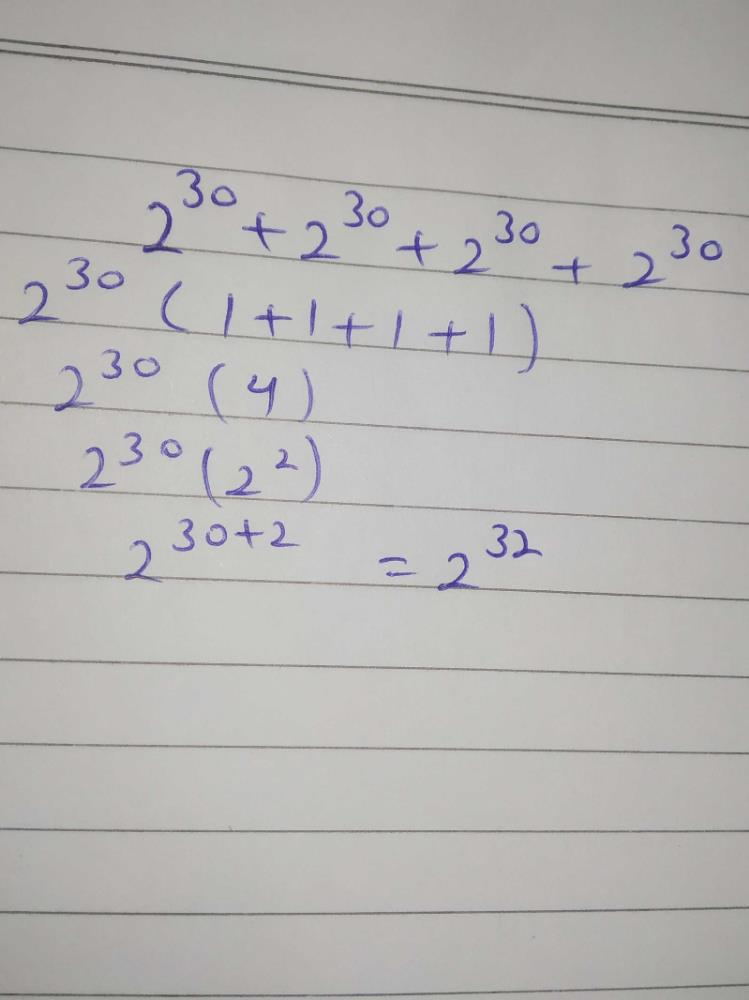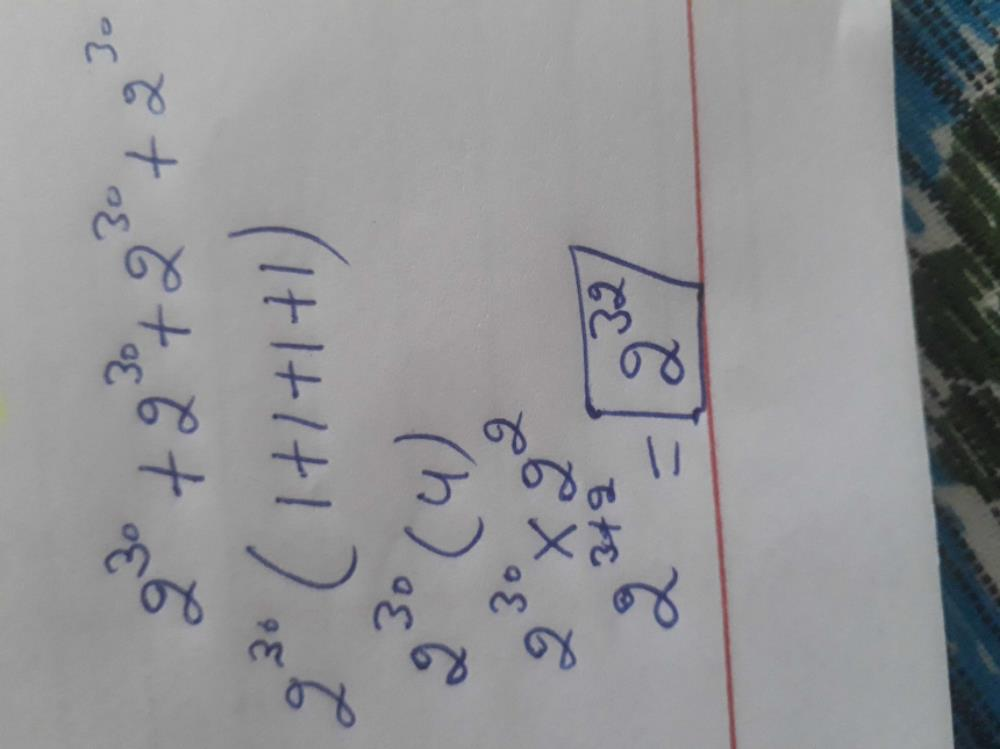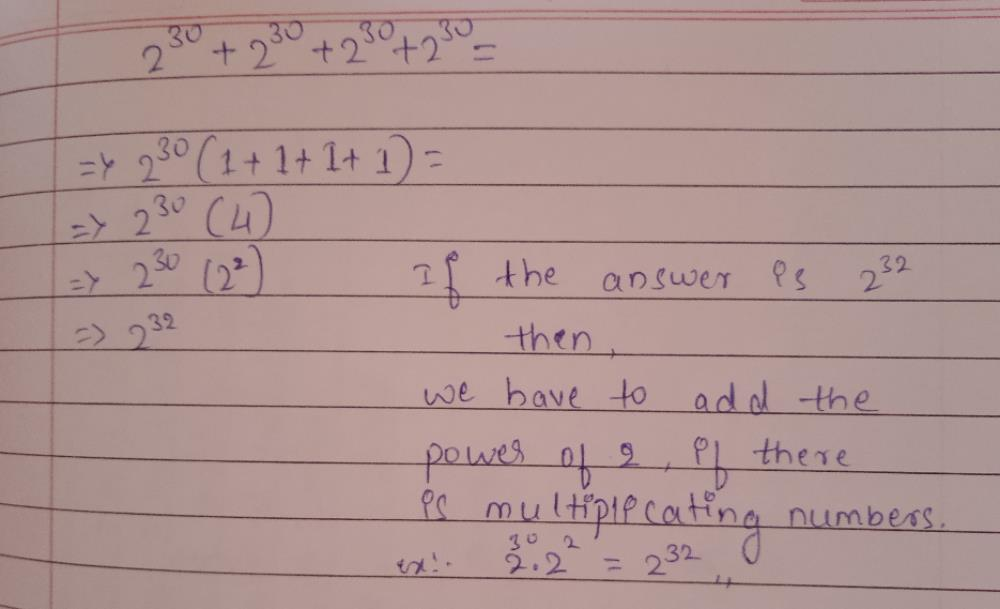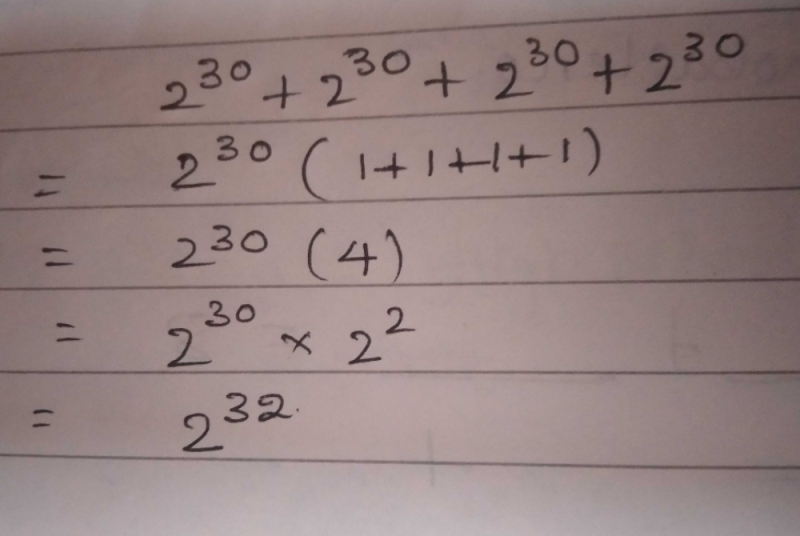Courses

# 230 + 230 + 230 + 230 =a)8120b)830 c)232 d)230 e)226Correct answer is 'C'. Can you explain this answer? Related Test: Problem Solving Practice Quiz - 1

## UPSC Question4 (2^30) = 2^2 ×2^30 = 2^32Sneha Music Jul 24, 2020Anushka Mehra May 28, 2020
I can't understand itNancy Redhu Jul 31, 2020Sakshi Giri Sakshi Jul 29, 2020
CC is correctFaisal Iqbal Shaikh Jul 22, 2020
BRangam Kumarranga Sep 16, 2020
2^30(1+1+1+1)=2^30(2^2)

=2^32
hence the option for that question is cRomeo Das Nov 23, 2020
4(2^30)=2^2×2^30=2^32Arsh Bhangu May 12, 2020
DDeepa Choudhary Aug 17, 2020
Yes the correct option is C i.e 2^32 as 2^30+2^30+2^30+2^30=2^30×(1+1+1+1)=2^30×4
2^30×2^2= (2)^30+2=2^32..
Hope u understand it....Gayatri Aware Aware Mar 21, 2021
Option c is correctSandeep Kumar Jul 02, 2020
4×(2^30)=2^2×2^30
= 2^32

* In multiplication if base are equal we can add powers

* In division if base are equal we can subtract the powers

example:

3^5/3^3 = 3^(5-3)= 3^2Arju Lathwal Lathwal Mar 10, 2021
Option C is correct.
2^30+2^30+2^30+2^30
=2^30(1+1+1+1)
=2^30(4)
=2^30×2^2
=2^(30+2)
=2^32Answer is 'B' because if base or exponent are same then add base and make exponent common for whole so answer would be 8 to the power 30.Bhavishay Gujjar Jul 23, 2020
2^30(1+1+1+1)
=2^30*(4)
=2^30*(2*2)
=(2^30)*(2^2)
=2^32Chhavi Tripathi Jun 21, 2020
Aap aise question kaha se late ho , please tell me.Sher Singh Jul 19, 2020
2^30+2^30+2^30+2^30 = 4×2^30 = 2^2 × 2^30 = 2^32Darshan Metgudmath Nov 24, 2020Thiraviyam Ramakrishnan Sep 13, 2020
Right ans is 'b'Rachit Jaiswal Sep 09, 2020
2^30 + 2^30 + 2^30 + 2^30

2^30(1+1) + 2^30(1+1)

2^30(2^1) + 2^30(2^1)

2^32Balwant Singh Mar 20, 2021
2^30 + 2^30 + 2^30 + 2^30

= 2^30 * (1+1+1+1) = 2^30 * 4       [take 2^30 common]

= 2^30 * 2^2                              [we can write 4 as 2^2]

= 2^(30+2) = 2^32                    [because a^b * a^c = a ^ (b+c) ]Sharukhan Kd Nov 07, 2020
2^30(1+1+1+1) =4×2^30=2^2×2^30=2^32Swapna Bathini Jul 30, 2020
4(2^30)=(2^2)(2^30)=2^2+30=2^32
when the bases are equal,we can add the powers(exponents)Ssssssss Ddd Jun 21, 2020
4(2^30)=2^2 * 2^30 =2^(30+2)= 2^32Janvhi Bachhav Aug 29, 2020
How could this plz explain itAjs Aug 03, 2020
The ans is CPrity Kumari Jul 08, 2020
2^30+2^30+2^30+2^30

2^30(1+1+1+1)

2^30×4

2^30×2^2 since, (a^m+a^n=a^m+n)

2^30+2

2^32 ans.Anagha Devi Prasad Jun 21, 2020
4(2^30) = 2^2(2^30)=2^(2+30)=2^32Jyoti Kumari Sep 16, 2020
AShamitha Sivakumar Sep 04, 2020Pragati Pathak Apr 30, 2020Kuldeep Bairagi Sep 13, 2020
Ans is (a)Sristi Singh Rajput Apr 19, 2020
C is right2³⁰+2³⁰+2³⁰+2³⁰
= 2³⁰×( 1+1+1+1)
= 2³⁰×4
=2³⁰×2²
=2³²Brain Games 3 weeks ago
4(2^30)= 8^30 OPTION B is correct.Hemaanshu Ranjan Nov 24, 2020
Take 2³⁰ common..its give you....2³⁰(1+1+1+1) add the you get 4*2³⁰and open 4 we get 2²*2³⁰ we know that .if the base are same in multiplication we can add their power.....then it becomes 2³² is an answer...Neeraj Barsiwal Jun 18, 2020
Vobdmas rule use thereRakesh Quinton Jul 27, 2020
Is it not 'd' the right answerPrakash Kumar Nov 19, 2020
2x4=8 '30×4=120Saili Shende Sep 03, 2020
=2^30(4)
=2^30(2^2)
=2^30+2
=2^32Mohammad Sameer Ansari Aug 12, 2020
4×2^30=2^2×2^30=2^(2+30)=2^32Davinderkaur Davinderkau Jul 29, 2020
A is right ansSidharth Gupta Mar 26, 2020
B is right onePrashant Shitole Jul 24, 2020
B is rightNancy 8130 Oct 05, 2020
(2+2+2+2)^30

so 8^30Kuldeep Singhal Jul 22, 2020
4(2^30)=2^2.2^30=2^32...Manjeer Singh Jul 29, 2020
8^120Niranjan Bujji Nov 16, 2020Nisarga H K Oct 01, 2020Bhargavi Satish Aug 27, 2020
2^30×2^2
2^32Vijay Billu Jul 09, 2020
Both option b and c are correct .Sanjay Kumar Aug 01, 2020
2^32Menka Chouhan Jan 02, 2021
'c'